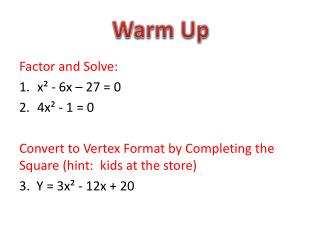DownloadDownload PresentationFactor and Solve: x² - 6x – 27 = 0 4x² - 1 = 0

# Factor and Solve: x² - 6x – 27 = 0 4x² - 1 = 0

Télécharger la présentation## Factor and Solve: x² - 6x – 27 = 0 4x² - 1 = 0

- - - - - - - - - - - - - - - - - - - - - - - - - - - E N D - - - - - - - - - - - - - - - - - - - - - - - - - - -
##### Presentation Transcript

1. Warm Up Factor and Solve: • x² - 6x – 27 = 0 • 4x² - 1 = 0 Convert to Vertex Format by Completing the Square (hint: kids at the store) 3. Y = 3x² - 12x + 20

2. Identify the 3 forms of a quadratic equation: Standard Format ax² + bx + c *** c is where the graph crosses the y axis *** Vertex Format y = a(x – h)² + k *** gives the vertex (h, k) *** Intercept Format y = a(x – p)(x – q) *** gives the roots, zeros or solutions of the graph ***

3. Write a quadratic equation in standard form that has the given solutions and passes through the given point. Which quadratic format is best to use given the roots of the graph? INTERCEPT FORM y=a(x – p)(x – q)

4. Step 1: Step 2: Use the other given point (-4, 3) to find A 3 = a (-4+1)(-4 + 3) Replace y With 3 Replace x With -4

5. A quadratic equation has roots of {-1, -3} and passes though (-4, 3). 3 = a (-4+1)(-4 + 3) Step 3: Step 4: